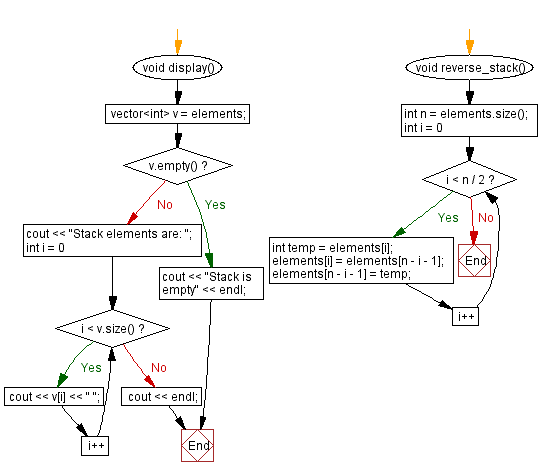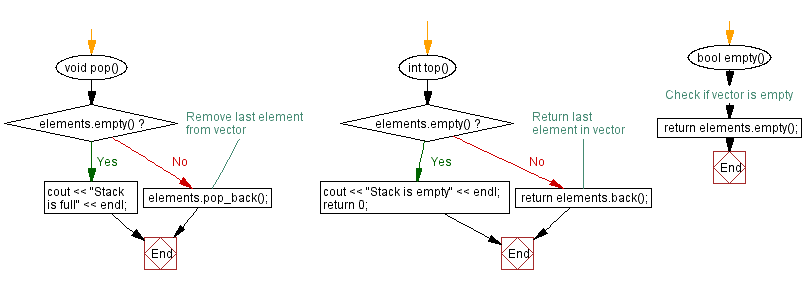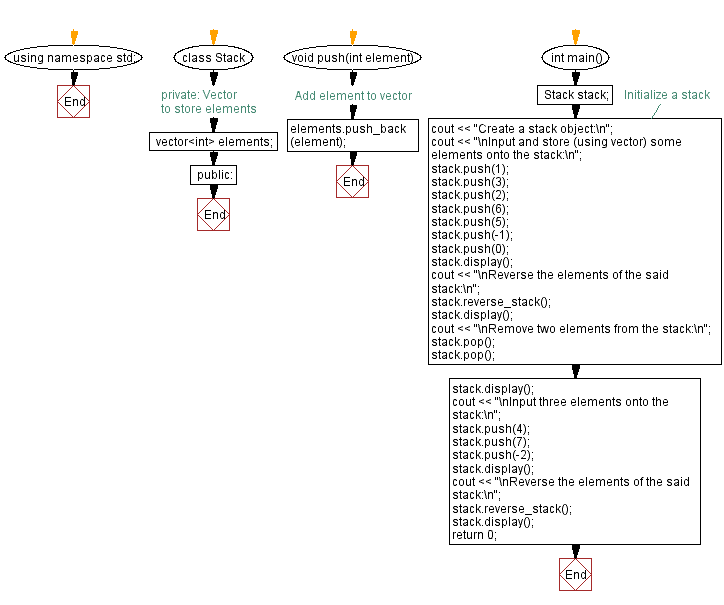﻿ C++ Reverse a stack (using a vector) elements

# C++ Stack Exercises: Reverse a stack (using a vector) elements

## C++ Stack: Exercise-26 with Solution

Write a C++ program that reverses the stack (using a vector) elements.

Test Data:
Create a stack object:
Input and store (using vector) some elements onto the stack:
Stack elements are: 1 3 2 6 5 -1 0
Reverse the elements of the said stack:
Stack elements are: 0 -1 5 6 2 3 1

Sample Solution:

C++ Code:

``````#include <iostream>
#include <vector>
#include<algorithm>

using namespace std;

class Stack {
private:
// Vector to store elements
vector<int> elements;

public:
void push(int element) {
elements.push_back(element);
}

void pop() {
if (elements.empty()) {
cout << "Stack is full" << endl;
} else {
// Remove last element from vector
elements.pop_back();
}
}

int top() {
if (elements.empty()) {
cout << "Stack is empty" << endl;
return 0;
} else {
// Return last element in vector
return elements.back();
}
}

bool empty() {
// Check if vector is empty
return elements.empty();
}

void display() {
vector<int> v = elements;
if (v.empty()) {
cout << "Stack is empty" << endl;
return;
}
cout << "Stack elements are: ";
for (int i = 0; i < v.size(); i++) {
cout << v[i] << " ";
}
cout << endl;
}

void reverse_stack() {
int n = elements.size();
for (int i = 0; i < n / 2; i++) {
int temp = elements[i];
elements[i] = elements[n - i - 1];
elements[n - i - 1] = temp;
}
}
};

int main() {
Stack stack;
//Initialize a stack
cout << "Create a stack object:\n";
cout << "\nInput and store (using vector) some elements onto the stack:\n";
stack.push(1);
stack.push(3);
stack.push(2);
stack.push(6);
stack.push(5);
stack.push(-1);
stack.push(0);
stack.display();
cout << "\nReverse the elements of the said stack:\n";
stack.reverse_stack();
stack.display();
cout << "\nRemove two elements from the stack:\n";
stack.pop();
stack.pop();
stack.display();
cout << "\nInput three elements onto the stack:\n";
stack.push(4);
stack.push(7);
stack.push(-2);
stack.display();
cout << "\nReverse the elements of the said stack:\n";
stack.reverse_stack();
stack.display();
return 0;
}
``````

Sample Output:

```Create a stack object:

Input and store (using vector) some elements onto the stack:
Stack elements are: 1 3 2 6 5 -1 0

Reverse the elements of the said stack:
Stack elements are: 0 -1 5 6 2 3 1

Remove two elements from the stack:
Stack elements are: 0 -1 5 6 2

Input three elements onto the stack:
Stack elements are: 0 -1 5 6 2 4 7 -2

Reverse the elements of the said stack:
Stack elements are: -2 7 4 2 6 5 -1 0
```

Flowchart:CPP Code Editor: